• ## 机器学习线性回归

千次阅读 2015-05-21 17:24:31
第一讲：线性回归 Supervised Learning 首先给出一些基本定义，x(i)x^{(i)} 表示输入变量或者输入特征，y(i)y^{(i)} 表示输出变量或者目标值。(x(i),y(i)(x^{(i)}, y^{(i}) 称为一对样本，一组样本 {(x(i),y(i));...
Supervised Learning

首先给出一些基本定义，x(i)<!--//--><![CDATA[//><!--
x^{(i)}
//--><!]]> 表示输入变量或者输入特征，y(i)<!--//--><![CDATA[//><!--
y^{(i)}
//--><!]]> 表示输出变量或者目标值。(x(i),y(i)<!--//--><![CDATA[//><!--
(x^{(i)}, y^{(i})
//--><!]]> 称为一对样本，一组样本 {(x(i),y(i));i=1,2,...,m}<!--//--><![CDATA[//><!--
\{(x^{(i)}, y^{(i)}); i=1,2,...,m\}
//--><!]]> 称为训练集，其中X<!--//--><![CDATA[//><!--
\mathbf{X}
//--><!]]> 表示输入变量的值域，Y<!--//--><![CDATA[//><!--
\mathbf{Y}
//--><!]]> 表示输出变量的值域，一般来说，我们都只考虑实数域。

The goal of supervised learning can be described as follows: given a training set, to learn a function h:X→Y<!--//--><![CDATA[//><!--
h: \mathbf{X} \rightarrow \mathbf{Y}
//--><!]]> so that h(x)<!--//--><![CDATA[//><!--
h(x)
//--><!]]> can predict the output value as close as the corresponding value of y<!--//--><![CDATA[//><!--
y
//--><!]]>. The function h<!--//--><![CDATA[//><!--
h
//--><!]]> is called hypothesis.

基于目标值的形式不同，Supervised Learning 主要分成两大类.

Regression Problem（回归问题）: 输出变量是连续值.  Classification Problem（分类问题）: 输出变量是离散值.

线性回归

我们先考虑一个简单的线性回归问题，我们假设输入变量 x<!--//--><![CDATA[//><!--
\boldsymbol{x}
//--><!]]> 是一个在二维空间R2<!--//--><![CDATA[//><!--
\mathbb{R}^2
//--><!]]>的向量， 我们有以下的表达式： hθ(x)=θ0+θ1x1+θ2x2<!--//--><![CDATA[//><!--
\begin{equation*}
h_\theta(\boldsymbol{x})=\theta_0+\theta_1x_1+\theta_2x_2
\end{equation*}
//--><!]]>θi<!--//--><![CDATA[//><!--
\theta_i
//--><!]]> 是 参数 (有时称为权值)，这些参数可以将输入域  X<!--//--><![CDATA[//><!--
\mathbf{X}
//--><!]]>  线性映射到输出域  Y<!--//--><![CDATA[//><!--
\mathbf{Y}
//--><!]]>. 我们引入截距的概念， 令 x0=1<!--//--><![CDATA[//><!--
x_0=1
//--><!]]>, 上述表达式可以改写成： hθ(x)=∑i=0nθixi=θTx<!--//--><![CDATA[//><!--
\begin{equation*}
h_\theta(\boldsymbol{x})=\sum_{i=0}^{n}\theta_ix_i=\boldsymbol{\theta}^T\boldsymbol{x}
\end{equation*}
//--><!]]>
现在，给出一组有 m<!--//--><![CDATA[//><!--
m
//--><!]]> 个样本的训练集，我们希望找到合适的参数 θ<!--//--><![CDATA[//><!--
\theta
//--><!]]>， 使得预测值 hθ(x)<!--//--><![CDATA[//><!--
h_\theta(x)
//--><!]]> 与目标值尽可能接近。为了估计参数 θ<!--//--><![CDATA[//><!--
\theta
//--><!]]>， 我们定义如下的 cost function: J(θ)=12∑i=1m(hθ(xi)−yi)2<!--//--><![CDATA[//><!--
J(\theta)=\frac{1}{2}\sum_{i=1}^{m}(h_\theta(\boldsymbol{x}^i)-y^i)^2
//--><!]]>
这个函数与最小均方误差函数很像，接下来，我们讨论如何求解参数 θ<!--//--><![CDATA[//><!--
\theta
//--><!]]>。

LMS Algorithm

为了求得最优的参数 θ<!--//--><![CDATA[//><!--
\theta
//--><!]]> 使得函数的均方误差最小，我们可以采用梯度下降算法进行求解，参数 θ<!--//--><![CDATA[//><!--
\theta
//--><!]]> 的更新可以
表示成如下： θj:=θj−α∂∂θjJ(θ)<!--//--><![CDATA[//><!--
\begin{equation*}
\theta_j: =\theta_j-\alpha \frac{\partial}{\partial \theta_j} J(\theta)
\end{equation*}
//--><!]]>
其中，α<!--//--><![CDATA[//><!--
\alpha
//--><!]]> 称为 Learning rate. 我们先给出函数的偏导数，为了简便起见，先考虑只有一对样本的情况 (x,y)<!--//--><![CDATA[//><!--
(\boldsymbol{x},y)
//--><!]]>. ∂∂θjJ(θ)=∂∂θj12(hθ(x)−y)2=2⋅12⋅(hθ(x)−y)⋅∂∂θj(hθ(x)−y)=(hθ(x)−y)⋅∂∂θj(∑i=0nθixi−y)=(hθ(x)−y)xj<!--//--><![CDATA[//><!--
\begin{equation*}
\begin{split}
\frac{\partial}{\partial \theta_j} J(\theta) & = \frac{\partial}{\partial \theta_j}\frac{1}{2}( h_\theta(\boldsymbol{x})-y)^2 \\
&  =2\cdot\frac{1}{2}\cdot( h_\theta(\boldsymbol{x})-y)\cdot\frac{\partial}{\partial \theta_j}( h_\theta(\boldsymbol{x})-y) \\
&  = (h_\theta(\boldsymbol{x})-y)\cdot \frac{\partial}{\partial \theta_j}(\sum_{i=0}^{n}\theta_i x_i-y) \\
&  = (h_\theta(\boldsymbol{x})-y)x_j \\
\end{split}
\end{equation*}
//--><!]]>
进而，我们可以得到只有一对样本时的更新准则： θj:=θj+α(yi−hθ(xi))xij<!--//--><![CDATA[//><!--
\begin{equation}
\theta_j: =\theta_j+\alpha (y^i-h_\theta(\boldsymbol{x}^i))x_{j}^{i}
\end{equation}
//--><!]]>
这就是有名的LMS更新原则，也叫Widrow-Hoff学习准则，参数 θ<!--//--><![CDATA[//><!--
\theta
//--><!]]> 更新的幅度取决于误差项的大小。从一对样本的情况，我们推导出参数θ<!--//--><![CDATA[//><!--
\theta
//--><!]]> 如何更新使得函数可以收敛。事实上，对于含有多个训练样本的情况，有两个方法可以对参数θ<!--//--><![CDATA[//><!--
\theta
//--><!]]> 进行更新，一个是 batch model， 另外一个是 stochastic model。

batch model的更新原则是每一次更新要遍历所有的样本，如下所示： Repeat until convergence{θj:=θj−α∑i=1m(yi−hθ(xi))xijfor every j}<!--//--><![CDATA[//><!--
\begin{equation*}
\begin{split}
& \text{Repeat until convergence} \qquad \{ \\
& \theta_j: =\theta_j-\alpha \sum_{i=1}^{m} (y^i-h_\theta(\boldsymbol{x}^i))x_{j}^{i}  \qquad   \text{for every $j$} \\
& \}
\end{split}
\end{equation*}
//--><!]]>而stochastic model每遇到一个样本就会进行一次更新，如下所示： Loop{for i=1 to m,{θj:=θj−α(yi−hθ(xi))xijfor every j}}<!--//--><![CDATA[//><!--
\begin{equation*}
\begin{split}
& \qquad \text{for $i=1$ to $m$,} \qquad \{  \\
& \qquad \theta_j: =\theta_j-\alpha (y^i-h_\theta(\boldsymbol{x}^i))x_{j}^{i}  \qquad   \text{for every $j$} \\
& \}
\end{split}
\end{equation*}
//--><!]]>从上面两种方式可以看出，batch model 每做一次更新要遍历所有的样本，这是非常耗时的，特别是在样本数非常多的时候，而stochastic model 是即时更新，每遇到一个样本就更新一次，通常情况下，stochastic model会下降地比batch model 快，不过stochastic model的一个缺陷是有可能无法收敛到全局最小值，而是在最小值附近来回扰动，这大概也是称之为stochastic model的原因，最终收敛的值带有一定的随机性，然而虽然只是收敛到全局最小值附近，很多时候这种近似已经非常靠近全局最小值，而且stochastic model的效率要更高，所以stochastic model一般会作为优先考虑的方法。

更通常的一种做法是结合两种方式，将整个训练集分割成很多个小的batch，然后利用stochastic model进行更新，每遇到一个小的batch，进行一次更新，这样做利用了stochastic model的高效，也在一定程度上减轻了在全局最小值附近的扰动。

参考文献

Andrew Ng, “Machine Learning”, Stanford University.

展开全文• 机器学习线性回归学习心得Data science with the kind of power it gives you to analyze each and every bit of data you have at your disposal, to make smart & intelligent business decisions, is ...
机器学习线性回归学习心得Data science with the kind of power it gives you to analyze each and every bit of data you have at your disposal, to make smart & intelligent business decisions, is becoming a must-have tool to understand and implement in your organization, it is very important that your business decisions are not based on intuition rather based on data analysis. 数据科学以其强大的功能使您能够分析您拥有的每一个数据，做出明智的业务决策，正成为了解和在您的组织中实施的必备工具，它非常重要的是您的业务决策不是基于直觉，而是基于数据分析。
Being a data science learner & practitioner, very often 经常成为数据科学学习者和实践者
我觉得： (I feel:)
“Data which you have in your repository is a gold mine, which needs to be harnessed with an intent to serve the humanity at large, as they are the key source of the same data. “ “您的存储库中的数据是一个金矿，需要利用它来为整个人类服务，因为它们是同一数据的关键来源。 “ Data has a story to tell. Being a data engineer and a business leader it’s your primary responsibility to treat them well, process it with an appropriate ML model, and build a solution that is relevant for both current and future user needs. With this intent, let’s begin our journey of understanding supervised ML using the Linear Regression model. 数据有故事可讲。 作为数据工程师和业务主管，您的首要责任是妥善处理它们，使用适当的ML模型进行处理，并建立与当前和将来的用户需求相关的解决方案。 出于这个目的，让我们开始使用线性回归模型了解监督ML的过程。
今日文章议程： (Agenda Of Today’s Article:)
What Is Supervised Machine Learning? 什么是有监督的机器学习？ Type Of Supervised Machine Learning? 监督机器学习的类型？ What Is Regression & Its Type? 什么是回归及其类型？ Understanding Linear Regression With Example? 通过示例了解线性回归？ Hands-On Labs Exercise On Linear Regression Using Python & Jupyter 动手练习使用Python和Jupyter进行线性回归  1.什么是监督学习？ (1. What Is Supervised Learning?)
监督学习： (Supervised Learning:)
In supervised learning, we are given a labeled data set(labeled training data) and the desired outcome is already known, where every pair of training data has some kind of relationship. 在监督学习中，我们得到了一个标记的数据集(标记的训练数据)，并且所需的结果是已知的，其中每对训练数据都有某种关系。
Supervised learning is where you have input variables (x) and an output variable (Y) and you use an algorithm to learn the mapping function from the input to the output. 监督学习是您拥有输入变量(x)和输出变量(Y)，并使用算法学习从输入到输出的映射函数的地方。
Y = f(X) Y = f(X)
The intent is to train the function so such an extent that whenever we have any new input data (x) you can easily predict the output variables (Y) for that given set of input data. 目的是对功能进行训练，以便在有任何新输入数据(x)时都可以轻松预测给定输入数据集的输出变量(Y)。
So here the training happens under the supervision of a teacher/assistant who already has the knowledge of correct answers and the algorithm iteratively makes predictions on the training data and is corrected by the supervisor. So when our learning algorithm achieves the acceptable level of training performance we put an end to the learning process. 因此，这里的培训是在已经具有正确答案知识的老师/助理的监督下进行的，并且该算法会反复对培训数据进行预测，并由主管进行纠正。 因此，当我们的学习算法达到可接受的训练水平时，我们就结束了学习过程。
监督式机器学习的类型： (Types Of Supervised ML:)
The most fundamental way one can categorize any supervised learning methodology is based on the type of problem statement it is trying to solve. At the high level, we can also say, what kind of business problem one is trying to solve using Supervised Machine Learning algorithms. 可以将任何监督学习方法分类的最基本方法是基于它要解决的问题陈述的类型。 从高层次上讲，我们还可以说，人们正在尝试使用监督式机器学习算法解决什么样的业务问题。
So, Within supervised machine learning we further categorize problems into the following categories: 因此，在有监督的机器学习中，我们将问题进一步分类为以下类别：
Regression 回归 Classification 分类  1.回归 (1. Regression)
Regression problems are the problems where we try to make a prediction on a continuous scale. Examples could be predicting the stock price of a company or predicting the temperature tomorrow based on historical data. Here temperature or sales parameters are continuous variables and we are trying to predict the change in sales value based on certain, given input variables like man-hours used, etc.. 回归问题是我们试图连续进行预测的问题。 例如，可以根据历史数据预测公司的股价或预测明天的气温。 这里的温度或销售参数是连续变量，我们试图根据某些给定的输入变量(例如使用的工时等)来预测销售价值的变化。
Regression is a method of modeling a target value based on independent predictors. This method is mostly used for forecasting and finding out the cause and effect relationship between variables. Regression techniques, mostly differ based on the number of independent variables and the type of relationship between the independent and dependent variables. 回归是一种基于独立预测变量对目标值建模的方法。 该方法主要用于预测和找出变量之间的因果关系。 回归技术大多基于自变量的数量以及自变量和因变量之间的关系类型而有所不同。
Regression Types : 回归类型：
Linear Regression 线性回归 Multiple Linear Regression 多元线性回归 Polynomial Regression 多项式回归 Decision Tree Regression 决策树回归 Random Forest Regression 森林随机回归 We will cover only Linear regression today and the rest we will cover later. 今天我们将只讨论线性回归，其余的将在以后讨论。
什么是线性回归？ (What Is Linear Regression?)
It is made up of two words Linear & regression. Let’s understand both before we get into the definition of linear regression 它由线性和回归两个词组成。 在进入线性回归的定义之前，让我们先了解一下
Linear: The word linear comes from the Latin word linearis, which means pertaining to or resembling a line 线性 ：线性一词来自拉丁语linearis，这意味着与线相似或相似
Regression: a kind statistical technique for estimating the relationships among dependent & independent variables. 回归 ：一种统计技术，用于估计因变量和自变量之间的关系。
Let’s combine them and define: 让我们结合起来并定义：
线性回归： (Linear Regression:)
It is a statistical approach to model between a dependent variable and one or more explanatory variables (or independent variables) to come up with a best fit linear line(linear equation, using least squared approach) represented in a most simplified manner as: 这是一种统计方法，可以在因变量和一个或多个解释变量(或自变量)之间建模，以最简化的方式表示最合适的线性线(线性方程式，使用最小二乘法)，其表示方式为：
Simple linear regression, 简单的线性回归
y =β0+β1X (y​=β0​+β1​X)
X=explanatory variables, X =解释变量，
β0​=y-intercept (constant term), β0 = y截距(常数)，
β1​=slope coefficients for the explanatory variable, β1 =解释变量的斜率系数，
We use linear regression to find the relationship between dependent & independent variables to find the best attribute (input variable)to use for model building in solving the regression type problems. 我们使用线性回归来找到因变量和自变量之间的关系，以找到最佳属性(输入变量)以用于解决回归类型问题的模型构建。
Linear Regression is further classified as 线性回归进一步分类为
Simple linear regression: It has only one explanatory variable 简单线性回归：只有一个解释变量 Multiple linear regression: It has more than one explanatory variable. Here multiple correlated dependent variables are predicted, rather than a single scalar variable(dependent variable) 多元线性回归：它具有多个解释变量。 这里预测了多个相关因变量，而不是单个标量变量(因变量) It represents line fitment between multiple inputs and one output, typically: 它表示多个输入和一个输出之间的线拟合，通常为：
多元线性回归的公式为 (The Formula for Multiple Linear Regression Is)
​yi​=β0​+β1​xi1​+β2​xi2​+…+βp​xip​+ϵ yi =β0+β1xi1+β2xi2+ ... +βpxip+ ϵ
where, for i=n observations:yi​=dependent variable 其中，对于 i = n个 观测值： yi =因变量
xi​=explanatory variables, xi =解释变量
β0​=y-intercept (constant term), β0 = y截距(常数)，
βp​=slope coefficients for each explanatory variable, βp =每个解释变量的斜率系数，
ϵ=the model’s error term (also known as the residuals)​ ϵ =模型的误差项(也称为残差)
In essence, multiple regression is the extension of ordinary least-squares (OLS) regression that involves more than one explanatory variable. 从本质上讲，多元回归是普通最小二乘(OLS)回归的扩展，涉及多个解释变量。
In linear regression, the relationships are modeled using linear predictor functions whose unknown model parameters are estimated from the data. 在线性回归中，使用线性预测函数对关系进行建模，这些函数的未知模型参数可从数据中估算出来。
Linear predictor functions: 线性预测函数：
In statistics and in machine learning, a linear predictor function is a linear function (linear combination) of a set of coefficients and explanatory variables (independent variables), whose value is used to predict the outcome of a dependent variable. 在统计学和机器学习中， 线性预测函数是一组系数和解释变量(独立变量)的线性函数(线性组合)，其值用于预测因变量的结果。
You will very often come across in our discussion the terms like dependent & independent variables, let’s try to understand the same so that our further discussion more sense going forward 在讨论中，您经常会遇到诸如因变量和自变量之类的术语，让我们尝试理解它们，以便我们进行进一步的讨论时更有意义
因变量： (Dependent Variables:)
In mathematical /statistical modeling the values of dependent variables depend on the values of independent variables. 在数学/统计建模中， 因变量的值取决于自变量的值。
The dependent variables represent the output or outcome whose variation is being studied. So in simplified terms 因变量表示正在研究其变异的输出或结果。 因此，简化而言
Whenever you try to predict any change in output variable based on any given input variable, this output variable is known as the dependent variable. We can also call them to be a target variable when we analyze them using a linear regression model. 每当您尝试基于任何给定的输入变量来预测输出变量的任何变化时，该输出变量都称为因变量。 当我们使用线性回归模型分析它们时，我们也可以称它们为目标变量。
自变量： (Independent variables:)
Also known in a statistical context as regressors, represent inputs or causes, that is, potential reasons for variation. This input variable which is largely mapped in the linear line equation to predict the possible outcome is known as dependent variables. 在统计上下文中也称为回归变量 ，代表输入或原因，即发生变化的潜在原因。 该输入变量主要映射在线性方程中，以预测可能的结果，称为因变量。
Example: Simple linear regression line(works for two variable) 示例：简单的线性回归线(适用于两个变量)
y= mX+C y = mX + C
Here y= dependent variable(Target variable) , 此处y =因变量(目标变量)，
X= Independent (input ) variable. X =独立(输入)变量。
It is best practice to represent the independent variable to be C=capital letter & dependent variable as a small letter. Though it’s not enforced. 最佳做法是将自变量表示为C =大写字母，并将因变量表示为小写字母。 虽然没有强制执行。
线性回归的关键概念： (Key Concepts Of Linear Regression:)
One needs to understand a few important concepts to make a better sense of the linear regression model which we will study going forward with example. So let’s cover them quickly 为了更好地理解线性回归模型，我们需要了解一些重要的概念，我们将通过示例进行研究。 因此，让我们快速覆盖它们
先决条件： (Prerequisites:)
To start with Linear Regression, you must be aware of a few basic concepts of statistics. i.e. 要开始使用线性回归，您必须了解一些统计学的基本概念。 即
Correlation (r): Explains the relationship between two variables, possible values -1 to +1 相关性(r)：说明两个变量之间的关系，可能的值是-1到+1 Variance (σ2): Measure of spread in your data 方差(σ2)：数据散布的量度 Standard Deviation (σ) : Measure of spread in your data (Square root of Variance) 标准偏差(σ) ：衡量数据传播的方差(方差的平方根) Normal distribution: Normal distribution, also known as the Gaussian distribution, is a probability distribution that is symmetric about the mean, showing that data near the mean are more frequent in occurrence than data far from the mean. In graph form, normal distribution will appear as a bell curve. 正态分布：正态分布，也称为高斯分布，是一种关于均值对称的概率分布，表明均值附近的数据比不均值的数据更频繁地出现。 在图形形式中，正态分布将显示为钟形曲线。 Residual (error term): Actual value(Which we have ) Minus Predicted value(Which came using linear regression ) 残差(误差项)：实际值( 我们有 )减去预测值(使用线性回归得出) To understand variance, standard deviation, normal distribution, please refer to my article below:https://www.mlanalytics.in/descriptive-statistics-fundamentals-for-data-science-aspirants/ 要了解方差，标准差，正态分布，请参阅以下我的文章： https : //www.mlanalytics.in/descriptive-statistics-fundamentals-for-data-science-aspirants/
Key Assumptions In the Linear Regression Model: 线性回归模型中的关键假设：
If we are building a linear regression model we need to take care of the following assumptions, in order to build an effective model that works well. 如果要构建线性回归模型，则需要考虑以下假设，以构建有效的模型。
The Dependent variable is continuous 因变量是连续的 There is a Linear relationship between Dependent Variable and Independent Variable. 因变量和自变量之间存在线性关系。 There is no Multicollinearity (no relationship between Independent variables 没有多重共线性(自变量之间没有关系) Residuals should follow Normal Distribution. 残差应遵循正态分布。 Residuals should have constant variance: Homoscedasticity 残差应具有恒定的方差：同方差 Residuals should be independently distributed/no autocorrelation 残差应独立分布/无自相关 To check the relationship : 要检查关系：
Between dependent and independent variable you can, 您可以在因变量和自变量之间
1. Perform Bivariate Analysis2. Calculate Variance Inflation factor: a value which is closer to 1 and till maximum 4 1.执行双变量分析2。 计算方差通货膨胀系数：该值更接近1，直到最大值4
To find whether residuals are normally distributed or not you can, 要确定残差是否为正态分布，可以，
Perform Histogram/ Boxplot 执行直方图/箱线图 Perform Kolmogorov Smirnov K’s test 进行Kolmogorov Smirnov K的测试  要检查同方差： (To check Homoscedasticity:)
You can Plot Residuals Vs. Predicted values and there should be no pattern in between them when you visualize them using data visualization tools. 您可以绘制残差对。 预测值，使用数据可视化工具对其进行可视化时，它们之间应该没有模式。 Perform the Non-Constant Variance Test. 执行非常数方差测试。  线性回归学习模型类型： (Linear Regression Learning Model Type:)
Simple Linear Regression: 简单线性回归： In simple linear regression when we have a single input, we can use statistics to estimate the coefficients. This requires that you calculate statistical properties from the data, such as means, standard, deviations, correlations, and covariance. 在只有一个输入的简单线性回归中，我们可以使用统计数据来估计系数。 这要求您从数据计算统计属性，例如均值，标准，偏差，相关性和协方差。
2.普通最小二乘法： (2. Ordinary Least Squares:)
When we have more than one input we can use Ordinary Least Squares to estimate the values of the coefficients. The Ordinary Least Squares procedure seeks to minimize the sum of the squared residuals. 当我们有多个输入时，我们可以使用普通最小二乘法来估计系数的值。 普通最小二乘程序试图最小化残差平方的总和。
It works by starting with random values for each coefficient. The sum of the squared errors is calculated for each pair of input and output values. A learning rate is used as a scale factor and the coefficients are updated in the direction towards minimizing the error. 它以每个系数的随机值开始。 为每对输入和输出值计算平方误差的总和。 学习率用作比例因子，并且朝着最小化误差的方向更新系数。
The process is repeated until a minimum sum squared error is achieved or no further improvement is possible. Here we select a learning rate (alpha) parameter that determines the size of the improvement step to take on each iteration of the procedure. We will look into it in detail later as this is out of scope for today’s article 重复该过程，直到获得最小平方和误差或无法进一步改善。 在这里，我们选择一个学习率(alpha)参数，该参数确定在该过程的每次迭代中采取的改进步骤的大小。 稍后我们将对其进行详细研究，因为这超出了本文的讨论范围
4.正则化： (4. Regularization:)
It is an extension to our linear model where we seek to both minimize the sum of the squared error of the model on the training data (using ordinary least squares) but also to reduce the complexity of the model (like the number or absolute size of the sum of all coefficients in the model). 这是对线性模型的扩展，在线性模型中，我们试图最小化模型在训练数据上的平方误差之和( 使用普通最小二乘法 )，同时降低模型的复杂度(例如模型的数量或绝对大小)模型中所有系数的总和)。
Two popular examples of regularization procedures for linear regression are: 线性回归的正则化程序的两个常见示例是：
Lasso Regression: where Ordinary Least Squares is modified to also minimize the absolute sum of the coefficients (called L1 regularization). 拉索回归(Lasso Regression) ：修改普通最小二乘以最小化系数的绝对和(称为L1正则化)。
Ridge Regression: where Ordinary Least Squares is modified to also minimize the squared absolute sum of the coefficients (called L2 regularization). 岭回归 ：对普通最小二乘进行修改以最小化系数的绝对和的平方(称为L2正则化)。
使用Jupyter和Python了解简单的线性回归： (Understanding Simple Linear Regression Using Jupyter & Python :)
We will use Jupyter notebook & do all mathematical calculations to plot the simple line of regression below, then will understand it all along the way. 我们将使用Jupyter笔记本并进行所有数学计算以在下面绘制简单的回归线，然后一路理解。
让我们开始吧 ： (Let’s Get Started :)
We will plot a scatter plot to visualize the given arrays x & y and then we will look into plotting a regression line, 我们将绘制散点图以可视化给定数组x和y，然后研究绘制回归线，
Execute the below-given code in your Jupyter notebook(i am assuming that you have already installed anaconda which comes pre-loaded with required python-support & Jupyter IDE) 在Jupyter笔记本中执行下面给出的代码(我假设您已经安装了预装了必需的python-support和Jupyter IDE的anaconda)
#Suppose we have the given value x & y  and there is a linear #relationship between both of them.import numpy as npimport pandas as pdimport numpy as npimport pandas as pdimport matplotlib.pyplot as pltimport seaborn as snsx = np.array([0, 1, 2, 3, 4, 5, 6, 7, 8, 9]) y = np.array([1, 3, 2, 5, 7, 8, 8, 9, 10, 12]) # number of observations/points n = np.size(x)#Lets plot a scatter plot for the given values colors = np.random.rand(n)area = 100 # 0 to 15 point radiiplt.scatter(x, y, area, colors, alpha=0.5)When you run the above code you will see the output as shown below: 运行上面的代码时，您将看到如下所示的输出：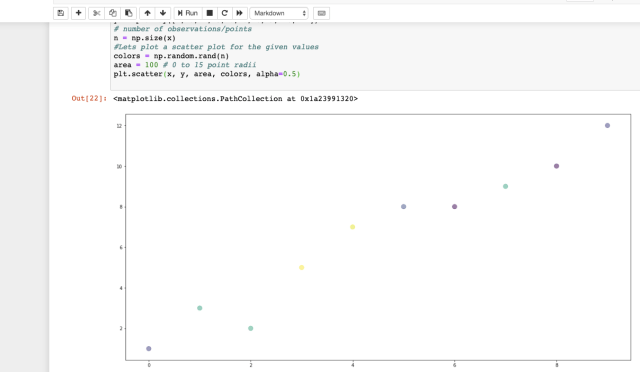最合适的生产线： (Best Fit Line :)
Now we need to find the line which fits best in the above scatter plot so that we can predict the response for any new feature values. (i.e a value of x not present in the dataset). This line is called the regression line. 现在我们需要找到最适合上述散点图的线，以便我们可以预测任何新特征值的响应。 (即数据集中不存在的x值)。 这条线称为回归线 。
The equation of the regression line is represented as: 回归线的方程表示为：
y = bo + b1X +∈ (y= bo + b1X + ∈)
这里， (Here,)
y represents the predicted response value for ith observation. y代表第i次观察的预测响应值 。 b0 and b1 are regression coefficients and represent y-intercept and slope of the regression line respectively. b0和b1是回归系数，分别代表y截距和回归线的斜率 。 ∈, is the residual error. ∈，是残差。 To build our simple linear regression model, we need to learn or estimate the values of regression coefficients b0 and b1. These coefficients will be used to build the model to predict responses. 要构建简单的线性回归模型，我们需要学习或估计回归系数b0和b1的值。 这些系数将用于构建模型以预测响应。
We will make use of the Least Squares technique to find the best fit line. 我们将利用最小二乘技术找到最佳拟合线。
Least squares is a statistical method used to determine the best fit line or the regression line by minimizing the sum of squares created by a mathematical function. The “square” here refers to squaring the distance between a data point and the regression line. The line with the minimum value of the sum of square is the best-fit regression line. 最小二乘法是一种统计方法，用于通过最小化由数学函数创建的平方和来确定最佳拟合线或回归线。 这里的“正方形”是指对数据点和回归线之间的距离进行平方。 平方和最小值最小的线是最佳拟合回归线。  步骤2：计算坡度和截距 (Step2: Calculating slope & intercept)
Let’s do some required calculation in python notebook to fins b0, b1. But before that, it is important to understand a few more formulas which we will perform in the python 让我们在python笔记本中进行一些必要的计算以查找b0，b1。 但在此之前，重要的是要了解我们将在python中执行的更多公式
#bo is intercept#b1 is slopeb0= (Σy)(Σx2) - (Σx)(Σxy)/ n(Σx2) - (Σx)2b1=(slope)= n (Σxy) - (Σx)(Σy) /n(Σx2) - (Σx)2Execute the below-given code in your Jupyter notebook to continue, 在Jupyter笔记本中执行下面给出的代码以继续，
#Step2 calculating slope & intercept #b0= (Σy)(Σx2) - (Σx)(Σxy)/ n(Σx2) - (Σx)2#b1=(slope)= n (Σxy) - (Σx)(Σy) /n(Σx2) - (Σx)2#mean of x and y vector m_x, m_y = np.mean(x), np.mean(y)# calculating cross-deviation and deviation about x SS_xy = np.sum(y*x) - n*m_y*m_x SS_xx = np.sum(x*x) - n*m_x*m_x # calculating regression coefficients b1 = SS_xy / SS_xx b0 = m_y - b1*m_x print("Coefficient b1 is: ",b1 )print("Coefficient b0 is: ",b0 )Run this code and you will see the output as given below: 运行此代码，您将看到如下输出：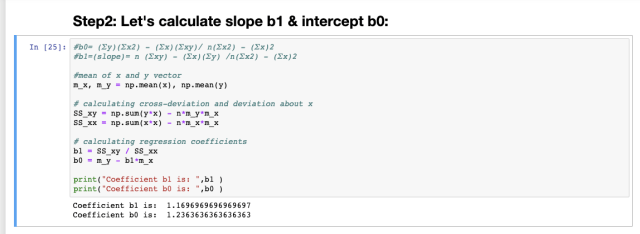So we have the required coefficient, b0= 1.23, b1= 1.16 因此我们有所需的系数b0 = 1.23，b1 = 1.16
第三步：绘制回归线： (Step3 : Plotting the line of regression:)
#Step 3 : Let's plot the scatter plot along with predicted y value #based on our slope & intercept#plotting the actual points as scatter plot plt.scatter(x, y, color = "m", marker = "o", s = 100) # predicted response vector y_pred = b0 + b1*x# plotting the regression line plt.plot(x, y_pred, color = "g") # putting labels plt.xlabel('x') plt.ylabel('y') #show plot plt.show()Execute the above code and run, you will see the output as given below in fig:4, 执行上面的代码并运行，您将看到如图4所示的输出，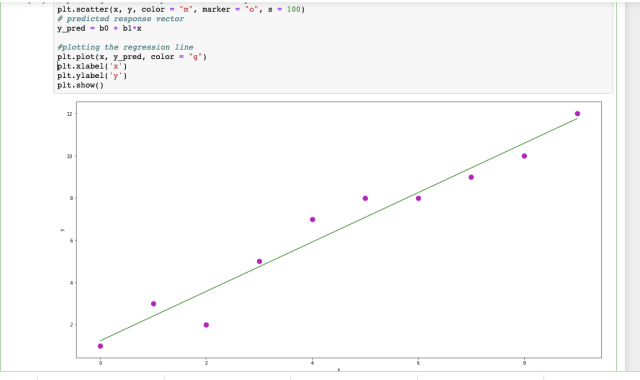步骤5：评估模型：使用R平方 (Step 5: Evaluating the model: Using R-Squared)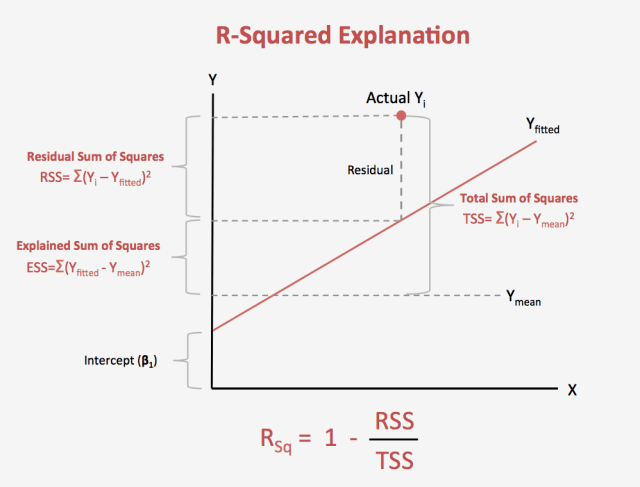Once we have the simple linear regression line( model ) we need to evaluate the same to measure its fitness. We will evaluate the overall fit of a linear model, using the R-squared value 一旦有了简单的线性回归线(模型)，我们就需要对其进行评估以衡量其适用性。 我们将使用R平方值评估线性模型的整体拟合
R平方： (R-Squared:)
R-squared is the proportion of variance explained R平方是解释的方差比例 It is the proportion of variance in the observed data that is explained by the model or the reduction in error over the null model 由模型解释的是观察数据中方差的比例，或者与原始模型相比误差的减少 The null model just predicts the mean of the observed response, and thus it has an intercept and no slope 零模型只是预测观察到的响应的平均值，因此具有截距且没有斜率 R-squared is between 0 and 1 R平方介于0和1之间 Higher values are better because it means that more variance is explained by the model. 值越高越好，因为这意味着模型可以解释更多的方差。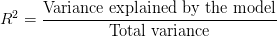使用Python计算R平方： (Calculating R-Squared Using Python:)
水平Y.mean()线 (Horizontal Y.mean() Line)
Next, we will place another line on our data. This is a key step in calculating our r-squared, as you will see in a minute. Write the below-given code and compile it 接下来，我们将在数据上放置另一行。 您将在稍后看到，这是计算r平方的关键步骤。 编写下面给出的代码并进行编译
#plot a horizontal line along mean of yline2 = np.full([m-x],[m_y])plt.scatter(x,y)plt.plot(x,line2, c = 'r')plt.show()The output is given below in fig:5 输出如下图：5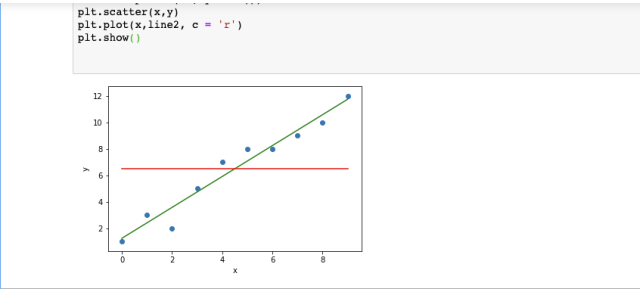Write the below given code and compile to get the r-squared value, 编写以下给出的代码并进行编译以获取r平方值，
differences_line1 = y_pred-yline1sum = 0for i in differences_line1: line1sum = line1sum + (i*i)line1sumprint(line1sum)differences_line2 = line2 — yline2sum = 0for i in differences_line2: line2sum = line2sum + (i*i)line2sumprint(line2sum)#Variance of our linear model: 5.624#Total variance of the target variable: 118.5diff = line2sum-line1sumprint(diff)rsquared= diff/line2sumprint(“R-Squared is : “, rsquared)#Let’s Verify The r-squared we calculated by using sklearn “r2_score” function:from sklearn.metrics import r2_scorer2Score = r2_score(y, y_pred)print(“Rsquared usinf sklearn: “, r2Score)#Observationprint("\nAs r-sqaured value is almost close to 1 , we can easily say that our linear regression model, y_pred = b0 + b1*x is a good fit linear regression line.")R-Squared value comes out to be: 0.95 R平方值得出为 ：0.95
测量R平方： (Measuring R-Squared:)
Higher values are better because it means that more variance is explained by the model. 值越高越好，因为这意味着模型可以解释更多的方差。
观察： (Observation:)
In our case, the r-squared value has come quite high to almost 0.95 very close to 1. So we can say that our model is better as it explains a larger variance in the data. 在我们的例子中，r平方值非常高，非常接近1，几乎达到0.95。因此，可以说我们的模型更好，因为它解释了数据中的较大差异。
R平方限制： (R-squared Limitations:)
You cannot use R-squared to determine whether the coefficient estimates and predictions are biased, which is why you must assess the residual plots. 您不能使用R平方来确定系数估计和预测是否有偏差，这就是为什么您必须评估残差图的原因。
Caution: R-squared does not indicate if a regression model provides an adequate fit to your data. A good model can have a low R2 value. On the other hand, a biased model can have a high R2 value! 注意 ：R平方不会指示回归模型是否可以完全适合您的数据。 好的模型可能具有较低的R2值。 另一方面，有偏差的模型可以具有较高的R2值！
So there is one another type of R2: adjusted R-squared and predicted R-squared. These two statistics address particular problems with R-squared. They provide extra information by which you can assess your regression model’s goodness-of-fit. We will cover this later. 因此，还有另一种R2：调整后的R平方和预测的R平方。 这两个统计数据解决了R平方的特定问题。 它们提供了额外的信息，您可以通过这些信息评估回归模型的拟合优度。 我们将在后面介绍。
Let’s understand the same example: 让我们理解相同的示例：
使用Sklearn Python库： (Using Sklearn Python Library:)
Here we can perform the same calculation to find the simple linear regression model using sklearn in just a few lines: 在这里，我们可以执行相同的计算，以使用sklearn在几行中找到简单的线性回归模型：
Here we go, 开始了，
import numpy as npimport pandas as pdimport matplotlib.pyplot as pltimport seaborn as snsfrom sklearn.linear_model import LinearRegressionx = np.array([0, 1, 2, 3, 4, 5, 6, 7, 8, 9]).reshape(-1,1) y = np.array([1, 3, 2, 5, 7, 8, 8, 9, 10, 12]) #invoke the LinearRegression function and find the bestfit model on #our given dataregression_model = LinearRegression()regression_model.fit(x, y) #this will give the best fit line # Let us explore the coefficients for each of the independent #attributesb1 = regression_model.coef_b0 = regression_model.intercept_print("b1 is: {} and b0 is: {}".format(b1, b0))plt.scatter(x, y, color = "m", marker = "o", s = 100) plt.plot(x, b1*x+b0)When you write & compile the above-given code snippet you will get the scatter plot with a line of regression, as shown below: 在编写和编译上述代码片段时，您将获得带有一排回归线的散点图，如下所示：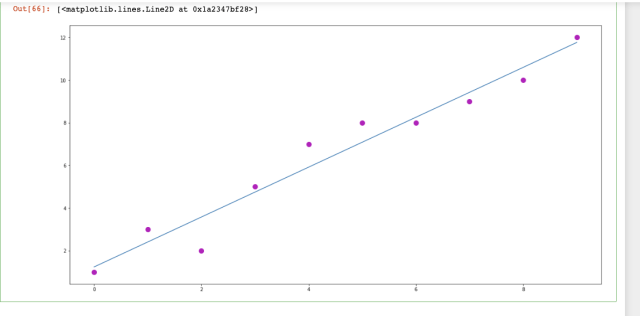让我们计算R2分数： (Let’s Calculate R2 Score:)
#sklearn has a function to calculate R-Squared value as seen #belowfrom sklearn.metrics import r2_score#y_pred is the predicted value which our linear regression model #predicted when we plotted the best fit line y_pred= regression_model.predict(x)r2Score = r2_score(y, y_pred) #here y is our original value print(r2Score)Output: 输出：
When you will compile the above code you will get the R-squared value to be 0.95 which we also calculated mathematically previously. 当您编译以上代码时，您将获得R平方值为0.95，这也是我们先前通过数学计算得出的。
加起来： (Summing Up:)
We covered the basics of simple linear regression and understood how we can find the linear regression model with one predictor value X. But there is more to the linear regression. We will not be often dealing with only one predictor value instead we will have large data sets with multiple independent values where you need to deal with multiple linear regression & polynomial type linear regression 我们介绍了简单线性回归的基础知识，并了解了如何找到具有一个预测值X的线性回归模型。但是线性回归还有很多。 我们将不会经常只处理一个预测值，而是会拥有包含多个独立值的大型数据集，您需要处理多个线性回归和多项式线性回归
下一步是什么？ (What’s Next?)
Will cover the following topics in the next part of Linear Regression, 在线性回归的下一部分中将涵盖以下主题，
Multiple linear regression model with one case study 多元线性回归模型与一个案例研究 polynomial regression model 多项式回归模型 Concept of underfitting & overfitting 欠拟合与过拟合的概念 Various techniques of error minimization in linear regression with examples 线性回归中的各种误差最小化技术与示例 Linear regression learning models like gradient descent, OLS, Regularization 线性回归学习模型，例如梯度下降 ， OLS ， 正则化 Thanks for being with me all along, will be back soon, keep loving keep sharing. 感谢您一直以来与我在一起，很快就会回来，继续爱着，继续分享。
翻译自: https://medium.com/swlh/linear-regression-explained-for-beginners-in-machine-learning-9e74f168d8a8机器学习线性回归学习心得
展开全文python 人工智能 深度学习
• 对于练习题的详细内容，和课程中推荐的octave编程实现，请见：吴恩达机器学习线性回归练习题：多变量线性回归（octave实现） 这边只贴出整个多变量线性回归步骤的Python实现代码，每个步骤在代码中有注释说明，变量...
练习题背景：网易云课堂->吴恩达机器学习课程->线性回归练习题->多变量线性回归

对于练习题的详细内容，和课程中推荐的octave编程实现，请见：吴恩达机器学习线性回归练习题：多变量线性回归（octave实现）

这边只贴出整个多变量线性回归步骤的Python实现代码，每个步骤在代码中有注释说明，变量和函数的命名也基本与练习题资料一致。

全部代码：

import pandas
import numpy
import matplotlib.pyplot as plt

def featureNormalization(X):
"""
数据标准化
:param X:
:return:
"""
mu = numpy.mean(X, axis=0)
# ddof的设置，会改变标准差的结算结果，因为总体误差和样本误差的计算公式不一样
sigma = numpy.std(X, axis=0, ddof=1)

X_norm = (X - mu) / sigma

return X_norm, mu, sigma

def computeCostMulti(X, y, theta):
"""
计算损失函数
:param X:
:param y:
:param theta:
:return:
"""
m = X.shape
costs = X.dot(theta) - y
total_cost = costs.transpose().dot(costs) / (2 * m)

def gradientDescentMulti(X, y, theta, alpha, iterNum):
"""
梯度下降实现
:param X:
:param y:
:param theta:
:param alpha:
:param iterNum:
:return:
"""
m = len(X)

J_history = list()

for i in range(0, iterNum):
costs = X.dot(theta) - y
theta = theta - numpy.transpose(costs.transpose().dot(X) * (alpha / m))

J_history.append(computeCostMulti(X, y, theta))

return theta, J_history

def learningRatePlot(X_norm, y):
"""
不同学习速率下的梯度下降比较
:param X_norm:
:param y:
:return:
"""
colors = ['b', 'g', 'r', 'c', 'm', 'y', 'k']
plt.figure()
iter_num = 50
# 如果学习速率取到3，损失函数的结果随着迭代次数增加而发散，值越来越大，不太适合在同一幅图中展示
for i, al in enumerate([0.01, 0.03, 0.1, 0.3, 1]):
ta = numpy.zeros((X_norm.shape, 1))
ta, J_history = gradientDescentMulti(X_norm, y, ta, al, iter_num)

plt.plot([i for i in range(len(J_history))], J_history, colors[i], label=str(al))

plt.title("learning rate")
plt.legend()
plt.show()

def normalEquation(X, y):
"""
正规方程实现
:param X:
:param y:
:return:
"""
return numpy.linalg.inv(X.transpose().dot(X)).dot(X.transpose()).dot(y)

if __name__ == '__main__':
# 读取数据
data_path = r'D:\ML\AndrewNg\machine-learning-ex1\ex1\ex1data2.txt'

# 切分特征和目标， 注意：索引是从0开始的
X = data.iloc[:, 0:2].values
y = data.iloc[:, 2:3].values

# 数据标准化
X_norm, mu, sigma = featureNormalization(X)

ones = numpy.ones((X_norm.shape, 1))

# 假设函数中考虑截距的情况下，给每个样本增加一个为1的特征
X_norm = numpy.c_[ones, X_norm]

# 初始化theta
theta = numpy.zeros((X_norm.shape, 1))

# 梯度下降学习速率为0.01
alpha = 0.01
# 梯度下降迭代次数为400
iterNum = 400

# 梯度下降
theta, J_history = gradientDescentMulti(X_norm, y, theta, alpha, iterNum)

# 画出梯度下降过程中的收敛情况
plt.figure()
plt.plot([i for i in range(len(J_history))], J_history)
plt.title("learning rate: %f" % alpha)
plt.show()

# 使用不同学习速率下的收敛情况
learningRatePlot(X_norm, y)

# 预测面积为1650，卧室数量为3的房子价格
x_pre = numpy.array([1650, 3])

x_pre_norm = (x_pre - mu) / sigma
numpy_ones = numpy.ones((1,))
x_pre_norm = numpy.concatenate((numpy.ones((1,)), x_pre_norm))
price = x_pre_norm.dot(theta)
print("通过梯度下降求解的参数预测面积1650、卧室数量3的房子价格为：%f" % price)

# 下面使用正规方程计算theta
X_ = numpy.c_[ones, data.iloc[:, 0:2].values]
y_ = data.iloc[:, 2:3].values

theta = normalEquation(X_, y)

# 预测面积为1650，卧室数量为3的房子价格
x_pre = numpy.array([1, 1650, 3])
price = x_pre.dot(theta)
print("通过正规方程求解的参数预测面积1650、卧室数量3的房子价格为：%f" % price)

代码执行结果：

通过梯度下降求解的参数预测面积1650、卧室数量3的房子价格为：289314.620338
通过正规方程求解的参数预测面积1650、卧室数量3的房子价格为：293081.464335

代码运行过程中会生成两张图：

迭代次数为400，学习速率为0.01时，梯度下降的收敛情况：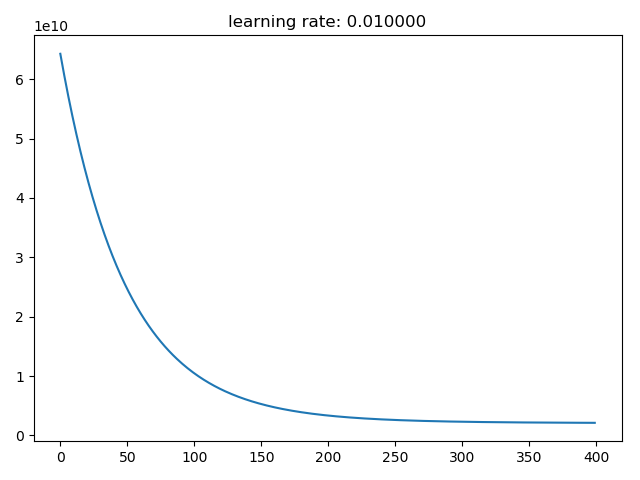迭代次数为50时，不同学习速率下梯度下降的收敛情况：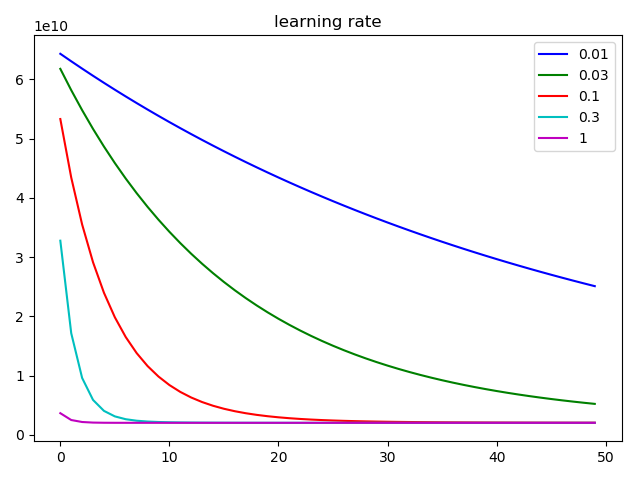展开全文吴恩达 编程题
• 简单线性回归，这是一种基于自变量值(X)来预测因变量值(Y)的方法。假设这两个变量是线性相关的。因此，我们尝试寻找一种根据特征或自变量(x)的线性函数来精确预测响应值(y)。步骤1：数据预处理更多说明可以去这篇...
简单线性回归，这是一种基于自变量值(X)来预测因变量值(Y)的方法。假设这两个变量是线性相关的。因此，我们尝试寻找一种根据特征或自变量(x)的线性函数来精确预测响应值(y)。步骤1：数据预处理更多说明可以去这篇文章看看：机器学习中的数据预处理步骤我们将按照之前的数据预处理信息图表那样来执行相同的步骤:导入相关库：其中第三相关库matplotlib是用来可视化数据的。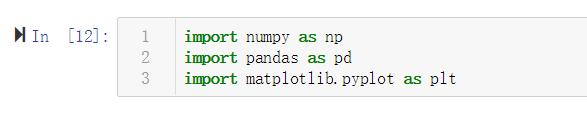导入数据集：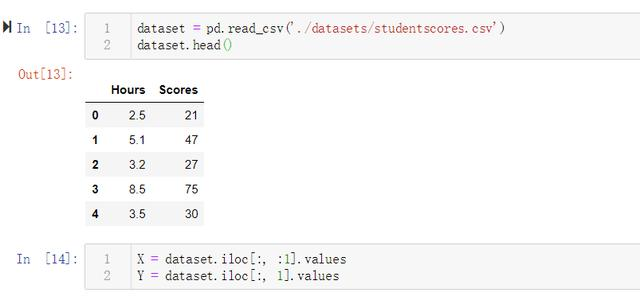检查缺失数据：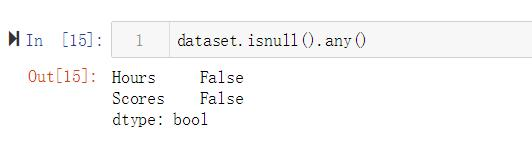从上面检查来看，未发现缺失值，所以这里不用做缺失值处理操作。划分数据集：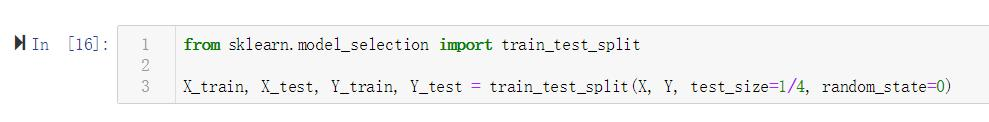特征缩放：由于这里的数据相对较均匀，差距落差没那么大，所以这里不需要对其进行数据缩放。步骤2：通过训练集来训练简单线性回归模型为了使用模型来训练数据集，我们将使用来自 sklearn.linear_model 库的 LinearRegression类,然后我们创建一个 LinearRegression 类的 regressor 对象最后我们将使用LinearRegression 类的 fit() 方法将 regressor 对象对数据集进行训练。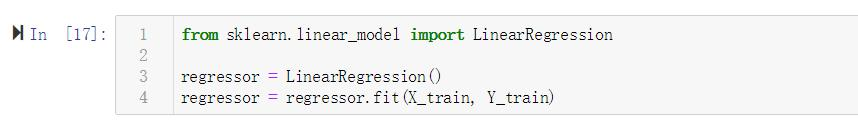步骤3：预测结果现在我们将预测来自测试集的观察结果。我们将把预测结果输出保存在向量 Y_pred 中。我们使用前一步中训练的回归模型 regressor 的 LinearRegression 类的预测方法来对结果进行预测。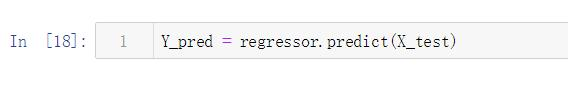步骤4：结果可视化最后一步是将结果可视化，我们将使用matplotlib.pyplot库对我们的训练集结果和测试集结果做散点图，以查看我们的模型预测效果。训练集结果可视化：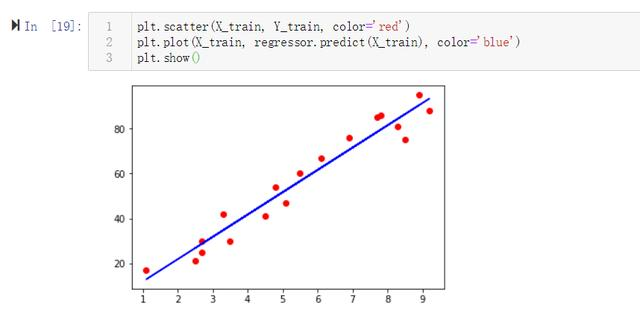测试集结果可视化：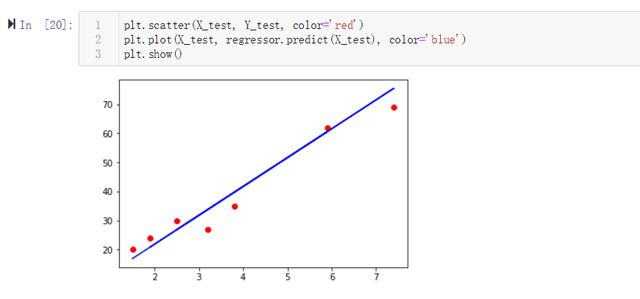展开全文• 机器学习 线性回归import numpy as np import matplotlib.pyplot as plt %matplotlib inlinex = np.linspace(1,10,20) a = np.random.randint(1,10,20)y = x * 4 + aplt.plot(x,y,'o')from sklearn.linear_model ...
•python 分析预测
• 文章目录机器学习 线性回归一、一元线性回归算法1.回归的理解2.回归应用3.线性回归（1）利用Sklearn做线性回归的预测（2）例：预测一组数据中当输入为12 对应输出的值。4.线性回归拟合原理(fit方法)（1）损失函数（2...
• 线性回归机器学习最基础的，也是最经典的算法，下面就来介绍这个算法。 假如我们要去银行贷款，那么银行会贷给我们多少钱，我们可以通过特征来计算出来。 数据：工资和年龄（2个特征） 目标：预测银行会贷款...
• LinearRegression(线性回归) 回归是一种解题的方法，或者说是“学习的方法”，也是机器学习中比较重要的概念。 回归一词的英文是Regression,单词原型regress大概的意思是“回退，倒退，退化”。 其实Regression回归...人工智能 数据分析 python
• 线性回归的损失函数 假设以波士顿房价为例子，真实的数据之间存在这样的关系 真实关系：真实房子价格 = 0.02×中心区域的距离 + 0.04×城市一氧化氮浓度 + (-0.12×自住房平均房价) + 0.254×城镇犯罪率 那么现在呢...python 深度学习 人工智能 数据分析
• 本文介绍机器学习中的线性模型的基本形式和求解线性回归方程方法 设问题为：判断一个同学对机器学习算法的掌握程度 有以下观点：1.数学基础 2.高级语言掌握程度 ...
• 线性回归的特征与目标的关系分析 线性回归当中主要有两种模型，一种是线性关系，另一种是非线性关系。在这里我们只能画一个平面更好去理解，所以都用单个特征或两个特征举例子。 线性关系 单变量线性关系 多变量...人工智能 python 深度学习 神经网络
•python
• 文章目录线性回归学习目标2.10 线性回归的改进-岭回归1 API2 观察正则化程度的变化，对结果的影响？3 波士顿房价预测 学习目标 掌握线性回归的实现过程 应用LinearRegression或SGDRegressor实现回归预测 知道回归...算法 python 深度学习
• https://www.laugh12321.cn/2019/01/02/evaluation_index_with_Linear_Regression/
• 单变量的线性回归模型就不再细说了，这里只记录多变量线性回归中几个需要实现的步骤和函数，其他内容在课程中的讲义和习题中都有详细描述。 特征标准化，对应featureNormalize函数 作用：消除不同特征间的量纲，...吴恩达 编程题
• 文章目录线性回归学习目标2.1 线性回归简介1 线性回归应用场景2 什么是线性回归2.1 定义与公式2.2 线性回归的特征与目标的关系分析 学习目标 掌握线性回归的实现过程 应用LinearRegression或SGDRegressor实现回归...人工智能 python 深度学习 算法...